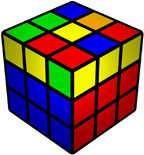# 1LLL

 1LLL methodInformation about the method Proposer(s): Bernard Helmstetter Proposed: 2000 Alt Names: OLLCP-EP Variants: ZBLL No. Steps: 1 No. Algs: 3915 Avg Moves: 12.58 HTM Purpose(s): Speedsolving, however the multitude of algorithms makes it much less useful in whole FMC

1 Look Last Layer means completing the last layer using only one look. On the 3x3x3, currently ZBLL is the only known practical system for achieving a 1-look last layer, but other experimental approaches such as 1-look 2-alg (such as M-CELL) may have potential.

A non-optimised database for all 1LLL algorithms & cases can be found at http://birdflu.lar5.com

## Number of 1LLL Cases

Assuming the last layer is an outer layer, the number of cases is calculated as:

corner orientations * edge orientations * corner permutations * edge permutations / 2

With the 2 at the end because of odd-even parity.

Numerically this is:

3^3 * 2^3 * 4! * 4! / 2 = 62208
• The 3^3 at the beginning is because there are 4 corners on the last layer while the final one depends on the remaining three.
• The 2^3 is because there are 4 edges on the last layer while the final one depends on the remaining three.

Treating cases which are the same, but rotated by 90, 180 or 270 degrees as the same case, the number of cases becomes:

62208/4 = 15552

Although these cases may be regarded as unique, some of them can be solved by applying the same algorithm from a different angle. For cases with no rotational symmetry, there are 3 equivalent cases which may be solved with the same alg. For cases with 180 degree rotational symmetry, there is 1 equivalent case which may be solved with the same alg. Cases with 90, 180 and 270 degree rotational symmetry are unique. By solving multiple cases with the same algorithm from different angles, the number of cases becomes:

3916 cases (including the solved state / AUF states and subsets such as PLL, ELL, 2GLL, L4C and ZBLL) which can be solved with 3915 algorithms

As well as rotationally symmetrical cases, there are also reflectively symmetrical cases. These may be solved by applying a reflection of the algorithm. Finally, some cases are inversions of others. These can be solved by reversing (inverting) the algorithm solving the original case. Exactly which cases are mirrors of each other requires a case-by-case analysis. Work by Bernard Helmstetter established that the number unique 1LLL cases (excluding mirrors and inverses) is:

1212 cases (including the solved / AUF states) which can be solved with 1211 algorithms

Since most speed solvers regard reflective symmetry and inversions as different cases (e.g. PLL is thought of as 21 algorithms rather than 13 algorithms plus mirrors and inverses), 1LLL should be thought of as 3915 algorithms.

PLL, ELL, and ZBLL are all subsets of 1LLL, albeit a small proportion of the algorithms. Knowing some of these subsets means less algorithms need to be learnt for a 1LLL:

• If the solver already knows all 21 PLL cases, which is a subset of ZBLL, there would be 3894 algorithms to learn.
• If the solver already knows all 493 ZBLL cases, there would be 3422 algorithms to learn.
• If the solver already knows all 29 ELL cases, there would be 3886 algorithms to learn.
• If the solver already knows all 518 ELL and ZBLL cases (4 EPLL cases appear in both sets), there would be 3397 algorithms to learn.

It should be noted that additional subsets of 1LLL also exist such as L3C (subset of L4C, which is a subset of ZBLL) , Pure OLL (orientation without affecting permutation), ZZLL (subset of ZBLL), ZZ-Blah (subset of ZBLL), Tripod LL and more.

## List of 1LLL subsetsThis page is under construction!One or more of our members are currently working on this page.Come back in a few days and it will hopefully be completed by then.